# A small guide to Random Forest - part 2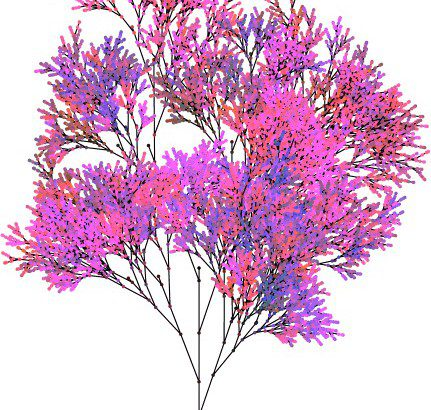This is the second part of a simple and brief guide to the Random Forest algorithm and its implementation in R. If you missed Part I, you can find it here.

#### randomForest in R

R has a package called randomForest which contains a randomForest function. If you want to explore in depth this implementation, I suggest to read the support webpage. Here I'd like to show the use of few parameters in the R function. I will here use the Titanic dataset from Kaggle to explore some functions and parameters in randomForest. The problem consists in predicting the survival of passengers, based on some data about them.

library(randomForest)
my_formula <- factor(Survived) ~ Sex + Pclass + Parch + SibSp + Embarked
my_forest <- randomForest(my_formula, data = train, ntree = 400, mtry = 3 )

Here I tuned the number of tree to grow with ntree (standard value is 500). The variable mtry specifies how many random features will be selected to grow a single tree. Here I chose mtry = 3, meaning that three features in the set {Sex, Pclass, Parch, SibSp, Embarked} will be randomly chosen every time a tree is grown. If I type:

my_forest

I get a briefing of the variables and the trained model:

Call:
randomForest(formula = my_formula, data = train, ntree = 400, mtry = 3)
Type of random forest: classification
Number of trees: 400
No. of variables tried at each split: 3
OOB estimate of error rate: 19.85%
Confusion matrix:
0 1 class.error
0 442 47 0.09611452
1 110 192 0.36423841

The OOB (out-of-bag) error is complementary to the accuracy, and it's here calculated as the ratio: . Look at the confusion matrix, which summarise how many cases were guessed right from our model. On the principal diagonal we can see the cases which are predicted well from my_forest. Indeed, we get:  which corresponds to the OOB error of . This is equivalent to saying that the accuracy of our model is . OOB error is calculated for each tree and you can access to such values by typing:

my_forest$err.rate Nerdy note: notice that the OOB error of the model is not the mean of my_forest$err.rate. They are calculated differently!
Another nice parameter is sampsize, meaning controlling how many rows of the dataframe will get selected to build a single tree:

my_forest <- randomForest(my_formula, data = train, ntree = 1000, mtry = 2, sampsize = (0.9*nrow(train)), replace=TRUE )

Here I asked that  of data is used for each tree. In addition, I set replace = TRUE, meaning that one row may be chosen more than once.

One nice aspect of randomForest is the variable importance, which can turn out very useful in feature engineering. If you type, for instance:

my_forest <- randomForest(my_formula, data = train, ntree = 400, importance = TRUE)
varImpPlot(my_forest)

you'll get a plot as follows.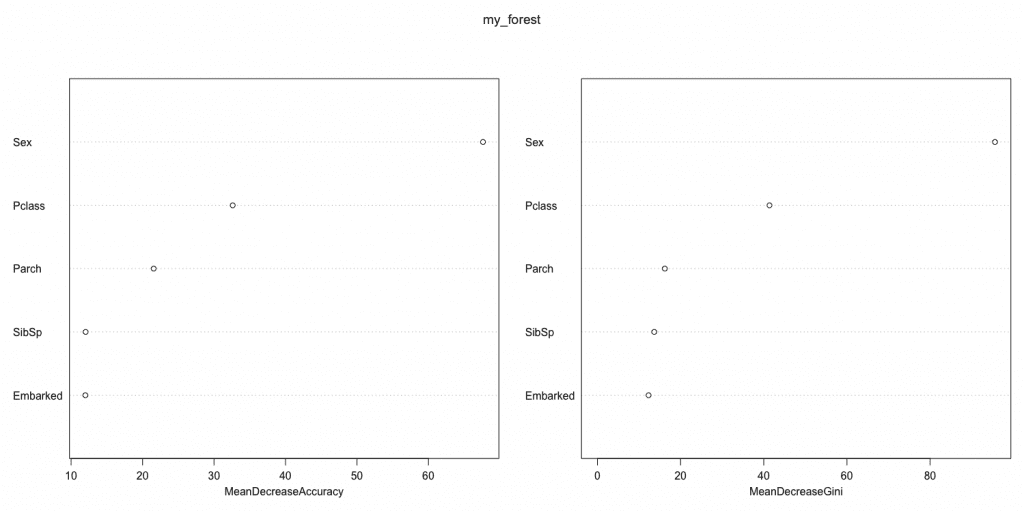The importance of each feature is measured in two ways, as described by documentation:

Here are the definitions of the variable importance measures. The first measure is computed from permuting OOB data: For each tree, the prediction error on the out-of-bag portion of the data is recorded (error rate for classification, MSE for regression). Then the same is done after permuting each predictor variable. The difference between the two are then averaged over all trees, and nor- malized by the standard deviation of the differences. If the standard deviation of the differences is equal to 0 for a variable, the division is not done (but the average is almost always equal to 0 in that case).

The second measure is the total decrease in node impurities from splitting on the variable, averaged over all trees. For classification, the node impurity is measured by the Gini index. For regression, it is measured by residual sum of squares.

Another possible choice is to set localImp = TRUE, and see how much each feature influenced the output of each single row. For example, here is the importance of Sex in our model: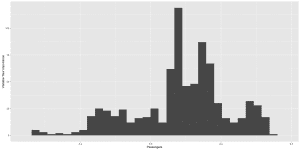#### Things to keep in mind

The R package randomForest allows to evaluate variable importance (in randomForest, set the parameter importance = TRUE, save the function output and pass it to varImpPlot()). However, keep in mind the following:

For data including categorical variables with different number of levels, random forests are biased in favor of those attributes with more levels. Methods such as partial permutations and growing unbiased trees can be used to solve the problem. (source: Wikipedia)

How many trees should one grow? In principle, the more the merrier. However, the information gain after a certain number is not worth the additional computational cost. The computational complexity of Random Forest is , where  is ntree,  is mtry and  is sampsize.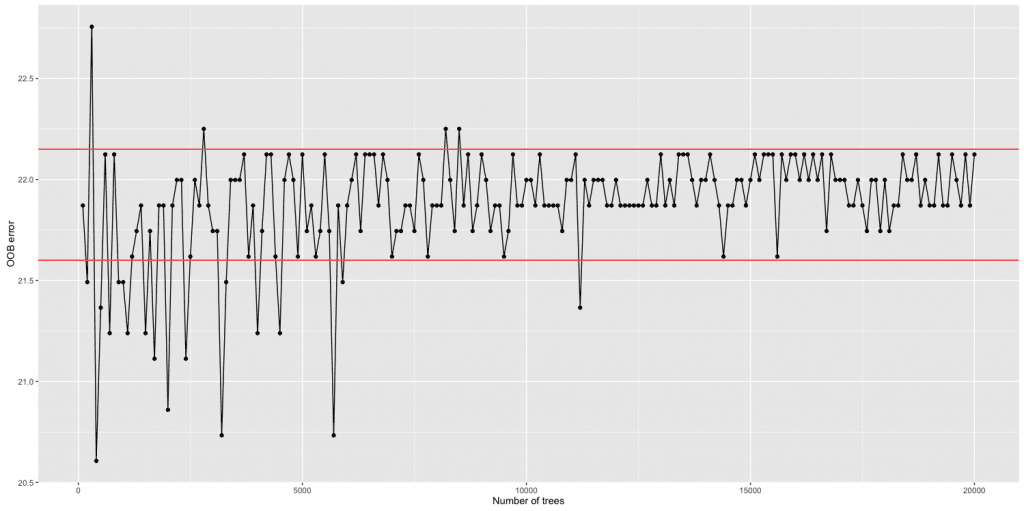I ran randomForest, changing ntree from 100 to 20 000, with a step of 100. After ntree = 5000 the OOB error has a 0.5% oscillation, showing that computational cost is too big compared to a gain in accuracy.

Another question is: how deep should I grow a tree? This is an interesting issue. Growing a superficial tree may lead to underfitting, while a too deep tree may cause overfitting. One idea to test the optimal value is to experiment with some very deep trees and observe how the accuracy behaves on its subsets.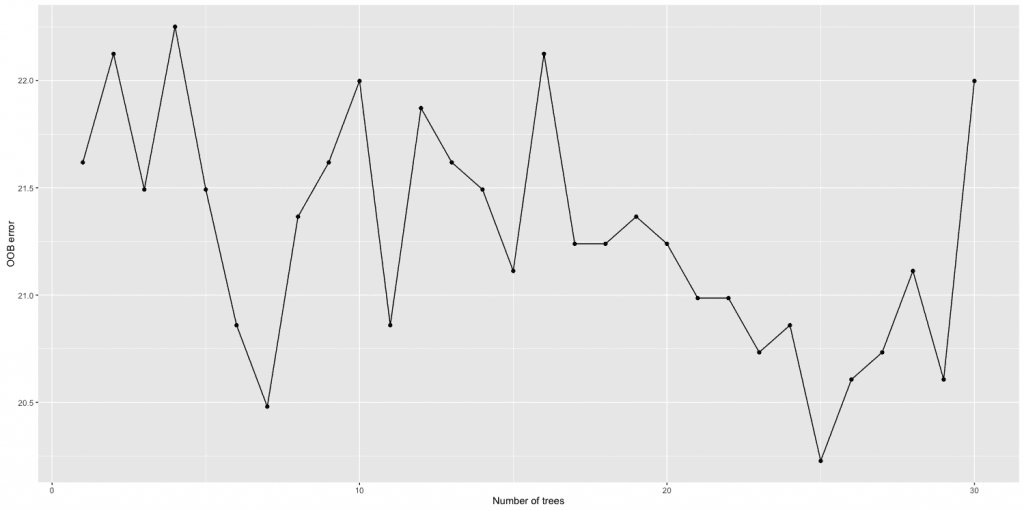Here I experimented with nodesize = 1, 2, ..., 30 and checked the corresponding OOB error. The minimum is attained at nodesize = 25. Given the oscillations, this is a less trivial choice than the number of trees, but experimenting takes less computational time.

The featured image was found on this webpage.#### Paola Elefante

Digital Scaling Project Manager at Plan International. Proud mother & wife. Shameless nerd&geek. Feminist. Undercover gourmet.

## One thought to “A small guide to Random Forest - part 2”

1.abel says:

Hi,
super tutorial!, but I want to know, what should I do to make graphs as the two at the end of this tutorial.

best regards
Abel

This site uses Akismet to reduce spam. Learn how your comment data is processed.